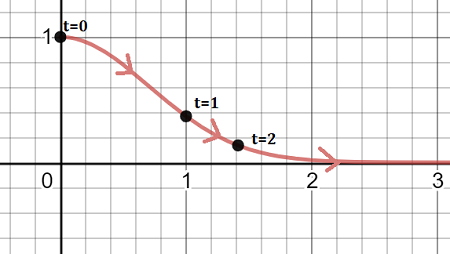# 1. Find the equation in x and y of the curve defined by the parametric equation...

## Question:

1. Find the equation in x and y of the curve defined by the parametric equation

{eq}\left\{\begin{matrix} x = t\\ y = \sqrt{t^2-2t+1} \end{matrix}\right. ,\;\; 0 \leq t \leq 4 {/eq}.

2. Find {eq}\frac{dy}{dx} {/eq} for

{eq}\left\{\begin{matrix} x = t^6 - 8t^2 + 2t\\ y = t^3 - 2t + 6 \end{matrix}\right. {/eq}

3. Find {eq}\frac{dy}{dx} {/eq} when {eq}x = t^6 + t^3 {/eq} and {eq}y = t^4 + t^2 {/eq}.

4. a) Sketch the graph of the curve {eq}x = \sqrt t, y = e^{-t}, t \geq 0 {/eq}.

b) Find an equation of the curve in rectangular coordinates.

c) Find the slope of the tangent line at t = 1.

## Parametric Equations

Instead of having {eq}y {/eq} written as a function of {eq}x {/eq}, we can consider both {eq}x {/eq} and {eq}y {/eq} as functions of a parameter {eq}t {/eq}, which often represents time. The graph of the parametric curve {eq}x=f(t) \hspace{0.1cm} y=g(t) {/eq} represents the movement of an object as {eq}t {/eq} increases. The tangent line to a parametric curve can be found by finding the ratio of the derivative of {eq}y {/eq} with respect to {eq}t {/eq} and the derivative of {eq}x {/eq} with respect to {eq}t {/eq}. That is, the slope of the tangent line is given by {eq}\dfrac{dy}{dx}=\dfrac{\frac{dy}{dt}}{\frac{dx}{dt}} {/eq}

## Question:

1. Find the equation in x and y of the curve defined by the parametric equation

{eq}\left\{\begin{matrix} x = t\\ y = \sqrt{t^2-2t+1} \end{matrix}\right. ,\;\; 0 \leq t \leq 4 {/eq}.

Since {eq}x=t {/eq}, simply substitute into the equation for {eq}y {/eq} to get {eq}y = \sqrt{x^2-2x+1}\\ y=\sqrt{(x-1)^2}\\ y=x-1 {/eq} for {eq}0\leq x\leq 4 {/eq}

2. Find {eq}\frac{dy}{dx} {/eq} for

{eq}\left\{\begin{matrix} x = t^6 - 8t^2 + 2t\\ y = t^3 - 2t + 6 \end{matrix}\right. {/eq}

{eq}\dfrac{dy}{dx} = \dfrac{\frac{dy}{dt}}{\frac{dx}{dt}}\\ \dfrac{dy}{dx} = \dfrac{6t^5-16t+2}{3t^2-2} {/eq}

3. Find {eq}\frac{dy}{dx} {/eq} when {eq}x = t^6 + t^3 {/eq} and {eq}y = t^4 + t^2 {/eq}.

{eq}\dfrac{dy}{dx} = \dfrac{\frac{dy}{dt}}{\frac{dx}{dt}}\\ \dfrac{dy}{dx}=\dfrac{4t^3+2t}{6t^5+3t^2}\\ \dfrac{dy}{dx} = \dfrac{4t^2+2}{6t^4+3t} {/eq}

4. a) Sketch the graph of the curve {eq}x = \sqrt t, y = e^{-t}, t \geq 0 {/eq}.

To sketch the curve, substitute values for {eq}t {/eq} in for {eq}x, y {/eq}b) Find an equation of the curve in rectangular coordinates.

Since {eq}x=\sqrt{t}\\ x^2=t {/eq}, we have {eq}y=e^{-t}\\ y=e^{-x^2}\\ {/eq}

c) Find the slope of the tangent line at t = 1.

{eq}\dfrac{dy}{dx} = \dfrac{\frac{dy}{dt}}{\frac{dx}{dt}}\\ \dfrac{dy}{dx} = \dfrac{-e^{-t}}{0.5t^{-0.5}}\\ \dfrac{dy}{dx} = \dfrac{-\sqrt{t}}{0.5e^t}\\ {/eq}

and so at t=1, we have the slope of the tangent line is

{eq}\dfrac{dy}{dx} = \dfrac{-\sqrt{1}}{0.5e^1}=-\dfrac{1}{0.5e} = -\dfrac{2}{e} {/eq}